Dedicated to those who never had the opportunity to receive a good education
Dedicated to those who never had the opportunity to receive a good education
0%

# The electric field of a point charge surrounded by a thick spherical shell

In the present post I will consider the problem of calculating the electric field due to a point charge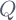surrounded by a conductor which has the form of a thick spherical shell. The conductor has zero net charge. The inner radius of the shell is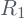, and the outer radius is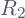. I will consider the case when the charge is at the center of symmetry of the spherical shell. I will discuss about the induced charges, and also about what happens when the shell is connected to the ground, and what happens when the point chargeis not at the center.

To solve the problem, let’s take a spherical Gaussian surface concentric with the shell. The area of the Gaussian surface is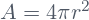. For, the Gauss Law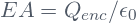immediately gives the answer: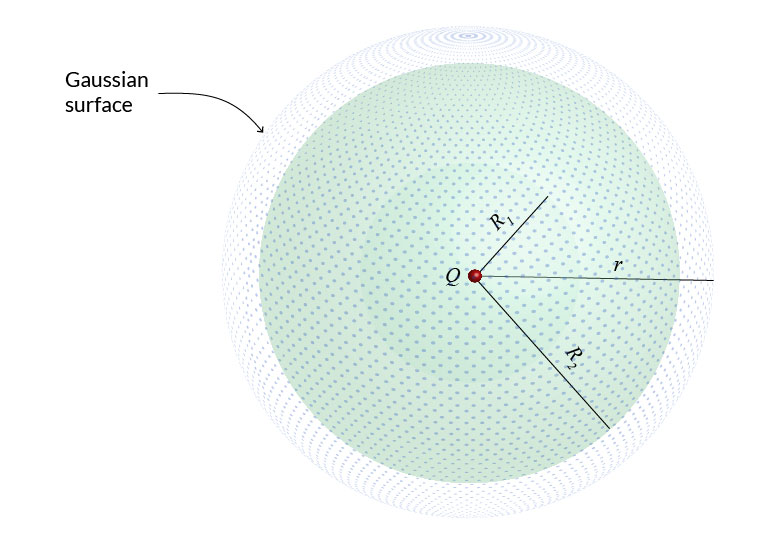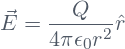Inside the conductor, i.e., for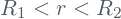, we know that the electric field is zero (This is one of the properties of conductors).

In the hollow part of the system, i.e., for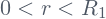, the Gauss Law leads to a similar result as for the field outside the shell: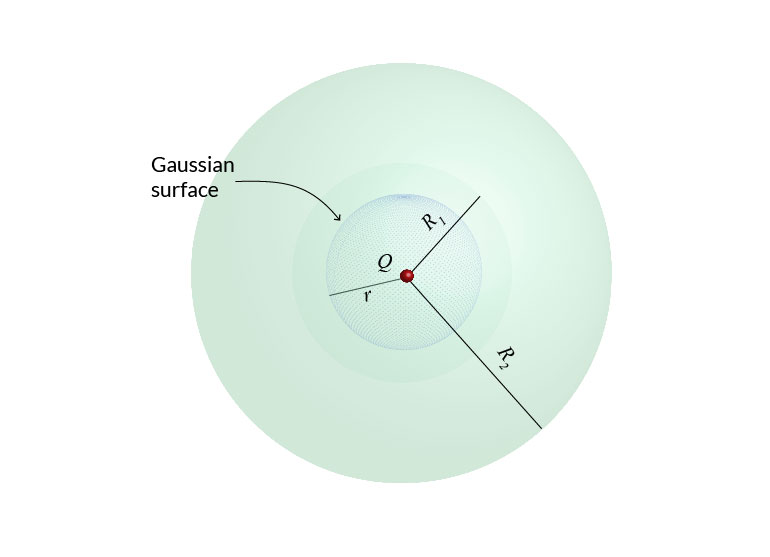We summarize the results as follows: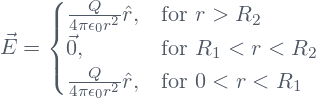The solution was easy, but, as we will see in what follow, we can learn some important things from this system.

## The induced charge on the shell

Let’s consider again the region inside the conductor, i.e., the region for. We know that there is no net charge in the volume occupied by the conductor, and that the electric field is zero in this region. If we take a spherical Gaussian surface with radius, the Gauss Law will  implie that the enclosed charge has to be zero. But the point chargelies at the center. Therefore, there must be an equal amount of charge, with opposite sign, i.e., a charge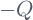, uniformly distributed on the inner surface of the shell. This charge is induced on the surface of the conductor by the point charge, and has a surface charge density given by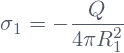But the conductor has zero net charge and there is no net charge in the volume occupied by the conductor. Therefore, there must be an amount of chargeuniformly distributed on the outer surface of the shell, with surface charge density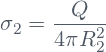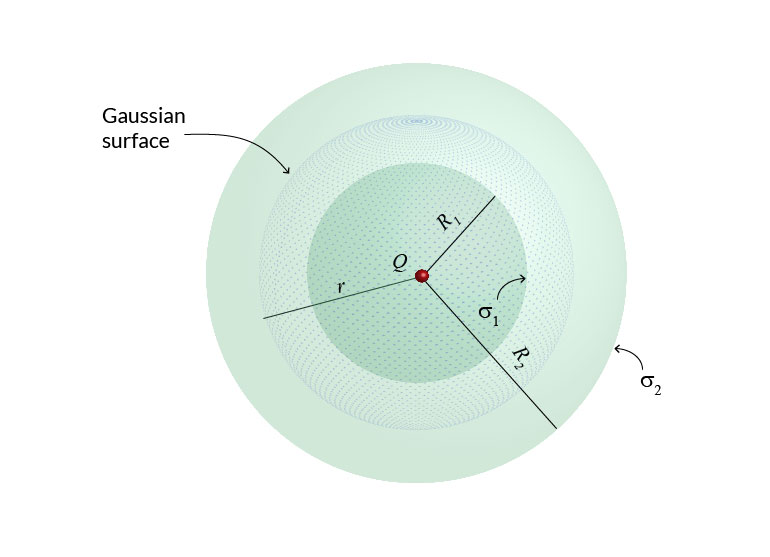We can say that the electric field outside the shell, is due to the surface charge density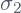. Curiously, if we displace the point chargefrom the center (without touching the conductor), the electric field in the hollow region, and the surface density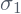at the inner radius will change, but the surface charge density, and the field in the exterior region will remain the same.

## The electric field after grounding the shell

The previous results have an additional consequence: If we connect the outer surface of the shell to the ground, then the electric field will be zero in the exterior region. I will explain how do we know that in the following section.

If the electric field in the exterior region is zero, then the Gauss Law, applied to a Gaussian surface surrounding the shell, implies that the total enclosed charge has to be zero. But the point chargeis at the center and an opposite chargeis distributed on the inner face of the shell. Therefore, the charge at the outer face of the shell has to be zero.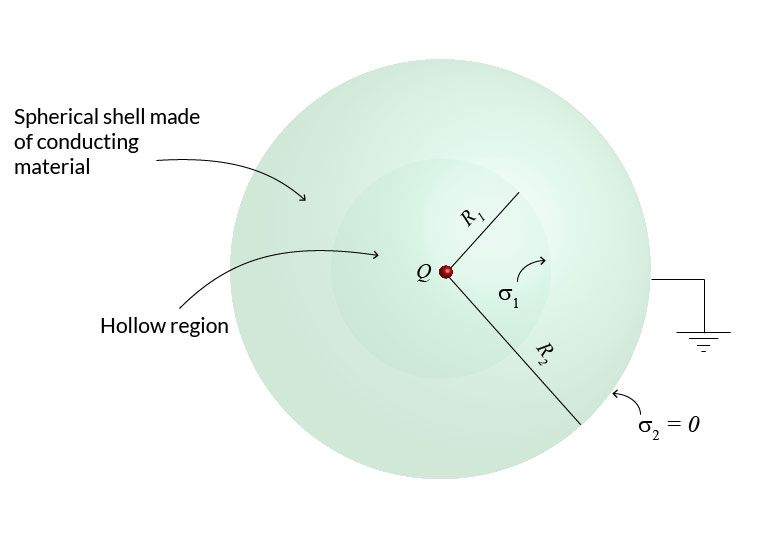Remember that before grounding the shell, the charge in the outer face was, and not zero. This means that an amount of chargewas transferred from the ground to the outer face of shell. Therefore, the conductor is not neutral anymore. It has acquired a net charge. This chargespreads uniformly on the outer face and neutralizes the charge that was present there before the grounding.

What will happen if now we disconnect the shell from the ground? The Electric field inside the conductor has to be zero all the time. Therefore the situation inside the conductor, at the inner surface, and in the hollow region will remain unchanged. But at the outer surface the net charge remains to be zero. Therefore, the Gauss Law, again implies that the electric field in the exterior region continues to be zero after the shell is disconnected from the ground. It is like saying that touching the ground turns off the external electric field.

## The electric field outside a grounded conductor is zero

How do we know that the electric field outside a conductor which is connected to the ground is zero? The answer is that experimental measurements show that this is so. But we can reach the same conclusion from the electromagnetic theory as we will see immediately.

After grounding the shell, it is easier to calculate first the electric potential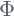in the outer region, an after that, to take the gradient of the potential in order to find the electric field according to the relation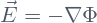.

The electric potential has to be a solution of the Lapalace equation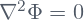, and in addition has to satisfy the boundary conditions at infinity and on the outer surface of the shell. The boundary condition at infinity is obviously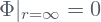.

The physical meaning of grounding a conductor is that we put it at zero electric potential. Therefore, the boundary condition at the outer face of the shell is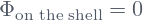.

Now it is easy to guess a solution, namely,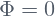. But there is a mathematical theorem which guaranties that there must be one, and only one solution to the Laplace equation that satisfies the appropriate boundary conditions. Therefore,is not just a solution, but is the only solution.

Thus, we conclude that both, the electric potential and the electric field, have to be zero outer the shell.

## What will happen if the point chargeis displaced from the center?

If the chargeis not at the center, we still can use the Gauss Law to calculate the electric field outside the shell because it remains spherically symmetric there. However, the electric field in the hollow part has not spherical symmetry anymore, and therefore, the Gauss law is not useful to find the field there.

In the hollow region, we have to calculate the electric potential first, and then to take its gradient. The electric potential in the hollow can be calculated  by using the method of images. I will show how to solve this problem in another post.

Share it!